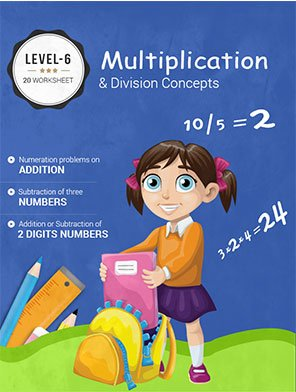\$5.00

## LEVEL 5 WORKSHEETS

The addition of Level 5 includes adding 2 digit numbers without regrouping and with regrouping to adding 3 digit numbers. Similarly, subtraction of this level includes subtracting numbers in horizontal and vertical form, and subtracting 3, 2, or 1 digit numbers from 3 digit numbers. Students are introduced to subtracting numbers ending at 0 or 0 at the middle, in Level 5.

Worksheet # Topics/Curriculum # of Pages 2pg Sample Add to cart
Unit-1 Understanding Patterns and Numbers to 1,000 10 pages\$1.00

Unit-2 Addition and Subtraction of Three Numbers 10 pages\$1.00

Unit-3 Adding 2 Digit Numbers with Regrouping 10 pages\$1.00

Unit-4 Adding 2 Digit Numbers: Sums Beyond 100 10 pages\$1.00

Unit-5 Adding Tens with Hundreds 10 pages\$1.00

Unit-6 Adding Ones with Hundreds 10 pages\$1.00

Unit-7 Subtracting Numbers in Horizontal Form 10 pages\$1.00

Unit-8 Subtracting Numbers in Vertical Form 10 pages\$1.00

Unit-9 Subtracting 2 Digit Numbers from 2 Digit Numbers 10 pages\$1.00

Unit-10 Addition or Subtraction of 2 Digit Numbers 10 pages\$1.00

Unit-11 Learning Numeration 10 pages\$1.00

Unit-12 Multiplication by 4 and 6 10 pages\$1.00

Unit-13 Multiplication by 7 to 9 10 pages\$1.00

Unit-14 Multiplying 2 Digit Numbers by 2, by 3, and by 4 10 pages\$1.00

Unit-15 Multiplying 2 Digit Numbers by 2 through 9 10 pages\$1.00

Unit-16 Multiplying 2 Digit Numbers Ending in 0 or 5 10 pages\$1.00

Unit-17 Multiplying 3 or More Digit Numbers by 1 Digit Numbers 10 pages\$1.00

Unit-18 Division without Remainders in Horizontal Form 10 pages\$1.00

Unit-19 Division with or without Remainders in Horizontal Form 10 pages\$1.00

Unit-20 Dividing the Numbers up to 60 by 2 through 9 10 pages\$1.00

Unit-21 Division without Remainders in Vertical Form 10 pages\$1.00

Unit-22 Dividing the Numbers up to 99 by 2 through 9 in Vertical Form 10 pages\$1.00

Unit-23 How to Tell Time 10 pages\$1.00

Unit-24 Identifying Fractions 10 pages\$1.00

Unit-25 Geometry 10 pages\$1.00